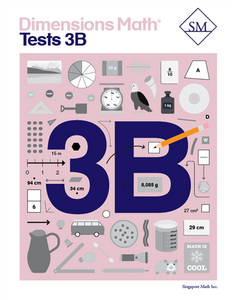# Dimensions Math Tests 3B

Vendor
Singapore Math Inc.
Regular price
\$13.70
Sale price
\$13.70
Quantity must be 1 or more

The Dimensions Math® PK-5 series is a new curriculum designed by Singapore Math Inc. to better serve U.S. teachers and students. Aspects of Singapore math curriculum have been updated for clarity and relevance, while preserving the solid foundation that makes it unique. The series follows the principles outlined in the Singapore Mathematics Framework and uses the Concrete > Pictorial > Abstract approach. The curriculum also incorporates Singapore mental math strategies and model drawing.

In the Dimensions Math program, concepts are introduced and explained in a new format with vibrant imagery. The implementation of this curriculum suits today’s needs, while the progression and scope that define Singapore math remain intact.

The Dimensions Math Tests books offer a series of assessment to help teachers and parents systematically evaluate student progress.

Samples of Dimensions Math Tests 3B

Chapter 8: Multiplying and Dividing with 6, 7, 8, and 9

Test A
Test B

Chapter 9: Fractions — Part 1
Test A
Test B

Chapter 10: Fractions — Part 2
Test A
Test B

Chapter 11: Measurement
Test A
Test B
Continual Assessment 1
Test A
Test B

Chapter 12: Geometry
Test A
Test B

Chapter 13: Area and Perimeter
Test A
Test B

Chapter 14: Time
Test A
Test B

Chapter 15: Money
Test A
Test B

Year-End Assessment
Test A
Test B